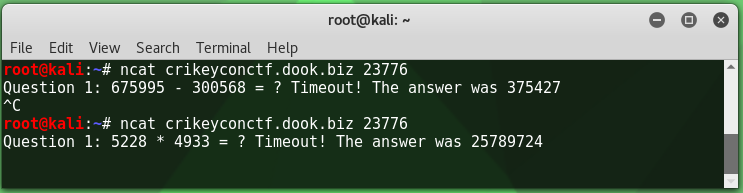# CrikeyCon 2017 - Fast Math WriteupMar 8, 2017
``````Category: Coding
Points: 300
Solves: 14
Description:  crikeyconctf.dook.biz:23776
``````

# Enumeration

Before doing anything else on this host I attempted to connect to it, receiving the following:The time between being presented with the challenge and receiving a timeout was a mere two seconds. Although handy with a calculator this wouldn’t be possible without a script/bot. I also noted that the response and timing to answer didn’t change on a second connection, but the base operator did. I then felt comfortable writing a script to connect to the host and return an answer to basic math questions (+-/*).

I put together a bot which would connect to the host and parse the challenge question using regex so it could answer it using an expression. This resulted in the following:

``````#!/usr/bin/python3

import socket
import re
import operator

MAXBUF = 4096
SENTINEL = 'flag'
CTF_BOT = ('crikeyconctf.dook.biz', 23776)

if __name__ == '__main__':
client = socket.socket(socket.AF_INET, socket.SOCK_STREAM)
client.connect(CTF_BOT)

while True:
data = b''

while True:
chunk = client.recv(MAXBUF)
data += chunk
if len(chunk) < MAXBUF:
break

# store decoded data for future usage
decoded = data.decode('utf-8')

#temporary
print(decoded)
#

# our flag likely contains flag{}, once it's revealed print received data and exit
if SENTINEL in decoded:
print(decoded)
break

match = re.search('[^\:\s]\d+.{3}\d+', decoded)

if not match:
raise ValueError("Invalid expression string")

expression = match.group(0)

# properly handle division
if '/' in expression:
expression = expression.replace('/', '//')

result = eval(expression)

# print results to screen to see script progress
print(expression + ' = ' + str(result))

# encode and transfer
data = str(result).encode('utf-8') + b'\n'
print('Sending: ' + str(result))
client.send(data)
``````

# Regular Expression (attempt)

The most important line here was the regex which consisted of the following (see if you can spot the mistake!):

``````match = re.search('[^\:\s]\d+.{3}\d+', decoded)
``````

This would skip everything proceeding the colon and whitespace and then group both sets of numbers, and the whitespace/operator between them for evaluation.

The response to this was the following:I spent far more time on this part of the challenge than I care to admit. I didn’t entirely read my logs and spent my time stuck on the two lines where I have Sending `1766845` and the response `459060` is not correct and put a lot of focus into trying to identify why a different response was being sent to what was calculated (not the truth, but it’s what I was thinking).

# Adding further regular expressions to catch x=x

Eventually I identified that the response to an answer was:

``````AnswerProvided is correct
``````

And then the next question would be presented. This was causing issues with my regex as I was passing values from the previous answer into my group, ultimately preventing the correct evaluation from sending.

I resolved this by continuing back to the beginning of my loop if there wasn’t a mathematical operator in my decoded string, putting this after the sentinel check as I didn’t want to miss my flag (which likely wouldn’t contain an operator). This looked like the following:

``````if not re.search('[-+/*]', decoded):
continue
``````

# Updating regular expression to handle more than one digit

This got me to question number 10 – at which point I realized that my regex from earlier:

``````match = re.search('[^\:\s]\d+.{3}\d+', decoded)
``````

Would only work if I were to be presented with a single digit question. I updated this to the following:

``````match = re.search('[^\:\s]\d+\d+.{3}\d+', decoded)
``````

Essentially the same as above, but it would now capture two digits over one as I didn’t expect the challenges to go past 99 (although could use \d* if they were to).

# Flag Reveal

After making the changes above and letting the bot run for fifty questions I was then rewarded with the flag (redacted):Success!

I hope this helped you to better understand how to approach this kind of programming based ctf item. If you’re Brisbane based, or find yourself here be sure to check out SecTalks.